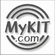L/C/F CalculatorThis formula calculates frequencies for any chosen value of capacitance and inductance...Enter a value for L and C to return Frequency.

Inductance (uH):

Capacitance (pF):

(MHz)

Frequency

This formula calculates inductance for any chosen value of frequency and capacitance...Enter a value for F and C to return Inductance.

Frequency (MHz):

Capacitance (pF):

(uH)

Inductance

This formula calculates capacitance for any chosen value of frequency and inductance...Enter a value for F and L to return Capacitance.

Frequency (MHz):

Inductance (uH):

(pF)

CapacitanceMyKIT 홈으로